SEARCH HOMEMath Central Quandaries & QueriesQuestion from Willie, a parent: Subtract and express the answer in simplest form. 5x-3/6-x+3/6Hi Willie,

I think this problem is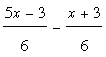.

Since the fractions have the same denominator we can write this as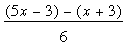. REmoving the parentheses and remembering to distribute the minus sign through the second set of parentheses yields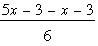. Now the numerator simplifies to become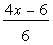. But we are not done yet since there is a common factor of 2 in both the numerator and denominator so finally I get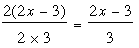.

I hope this helps,
PennyMath Central is supported by the University of Regina and The Pacific Institute for the Mathematical Sciences.